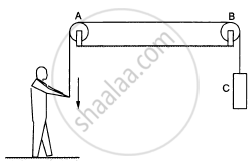Department of Pre-University Education, KarnatakaPUC Karnataka Science Class 11

# List All the Forces Acting on (A) the Pulley A, (B) the Boy and (C) the Block C in Figure. - Physics

Short Note

List all the forces acting on (a) the pulley A, (b) the boy and (c) the block C in figure.#### Solution

Forces acting on the pulley A :

1. A tension exerts electromagnetic force between the string and pulley A.
2. A pair of gravitational force between the Earth and pulley.

Forces acting on the boy :

1. A tension exerts electromagnetic force between the string and the boy.
2. A pair of gravitational force between the Earth and the boy.

Forces acting on the block C :

1. A tension exerts electromagnetic force between the string and block C.
2. A pair gravitational force between block C and the Earth.
Concept: Work Done by a Constant Force and a Variable Force
Is there an error in this question or solution?

#### APPEARS IN

HC Verma Class 11, Class 12 Concepts of Physics Vol. 1
Chapter 4 The Forces
Short Answers | Q 8 | Page 61
Share